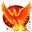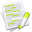#wx.lib.floatcanvas.FCObjects.PointSet¶

Draws a set of points

If Points is a sequence of tuples: Points[N] is the x-coordinate of point N and Points[N] is the y-coordinate.

If Points is a NumPy array: Points[N,0] is the x-coordinate of point N and Points[N,1] is the y-coordinate for arrays.

Each point will be drawn the same color and Diameter. The Diameter is in screen pixels, not world coordinates.

The hit-test code does not distingish between the points, you will only know that one of the points got hit, not which one. You can use PointSet.FindClosestPoint(WorldPoint) to find out which one

In the case of points, the HitLineWidth is used as diameter.

##Class Hierarchy¶Inheritance diagram for class PointSet:

##Methods Summary¶

 __init__ Default class constructor. DrawD2 FindClosestPoint Returns the index of the closest point to the point, XY, given SetDiameter Sets the diameter

##Class API¶

class PointSet(PointsObjectMixin, ColorOnlyMixin, DrawObject)

Draws a set of points

If Points is a sequence of tuples: Points[N] is the x-coordinate of point N and Points[N] is the y-coordinate.

If Points is a NumPy array: Points[N,0] is the x-coordinate of point N and Points[N,1] is the y-coordinate for arrays.

Each point will be drawn the same color and Diameter. The Diameter is in screen pixels, not world coordinates.

The hit-test code does not distingish between the points, you will only know that one of the points got hit, not which one. You can use PointSet.FindClosestPoint(WorldPoint) to find out which one

In the case of points, the HitLineWidth is used as diameter.

### Methods¶

__init__(self, Points, Color="Black", Diameter=1, InForeground=False)

Default class constructor.

Parameters
• Points – takes a 2-tuple, or a (2,) NumPy array of point coordinates

• Color – see SetColor

• Diameter (integer) – the points diameter

• InForeground (boolean) – should object be in foreground

DrawD2(self, dc, Points)

FindClosestPoint(self, XY)

Returns the index of the closest point to the point, XY, given in World coordinates. It’s essentially random which you get if there are more than one that are the same.

This can be used to figure out which point got hit in a mouse binding callback, for instance. It’s a lot faster that using a lot of separate points.

Parameters

XY – the (x,y) coordinates of the point to look for, it takes a 2-tuple or (2,) numpy array in World coordinates

SetDiameter(self, Diameter)

Sets the diameter

Parameters

Diameter (integer) – the points diameter